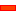﻿ MechDesigner Reference & User Interface > Dialog-Boxes > Dialog: Function-Block: Motion-Path

# Dialog: Function-Block: Motion-Path

Navigation:  MechDesigner Reference & User Interface > Dialog-Boxes >

# Dialog: Function-Block: Motion-Path

## Motion-Path FB

A Motion-Path FB adds (one ore more) Motion-Points to a sketch-path.

Each Motion-Point moves along the sketch-path when you connect a wire (with motion-data) to the input-connector of the Motion-Path FB.

The Data-Type at the input to the Motion-Path FB is important. When the Data-Type at the input to the Motion-Path FB are:

Linear Units (mm), then the:

Distance the Motion-Point(s) moves along the sketch-path = Input Motion-Value (units)

Angular Units (degrees), then the:

Distance the Motion-Point(s) moves along the sketch-path = Input Motion-Value (degrees) /360 (units)

Note:

A Sketch-Path is one or more sketch-elements that you join end-to-end with Merged-Points. Sketch-Paths for a Motion-Path FB are:

Open(Γ), or

Closed(Δ), or

Crossed(α), or

Not crossed(ε).

### Open Motion-Path FB dialog-boxEdit the Motion-Path FB:

 1.Double-click the Motion-Path FB in the graphic-area - or - 1.

The Motion-Path dialog-box is now open.

Motion-Path dialog-boxMotion-Path dialog-boxPoint-Parameters tabLength Control tab - Path Length using Diagram

### About Motion-Range and Output Data Type from a Motion FB

The motion of the Motion-Point along the sketch-path is a function of the Data-Type at the input-connector to the Motion-Path FB: Does the data-type have Linear or Rotary units?

When you connect a wire from the output of a Motion FB you must also set the Output Data Type with the Motion FB dialog-box (see Motion FB dialog-box).

Motion FB > Output Data Type

There are three Output Data Types

Motion - do not use with a Motion-Path FB

Linear - see below

Rotary - see below

Motion FB > Output Data-Type - Linear

 The position of the Motion-Point is scaled on a 1 : 1 basis with the motion-value at the output-connector of the Motion FB and input-connector its Motion-Path FB ∴ the position of the Motion-Point along the sketch-path = motion-value at input-connector in linear units.

Motion FB > Output Data-Type - Rotary

 The sketch-path has a scale of 0 to 360. A motion-value at the input of 360 would move the Motion-Point the complete extent of the sketch-path. ∴ the position of the Motion-Point along the sketch-path = motion-value at input-connector × total length of the sketch-path / 360